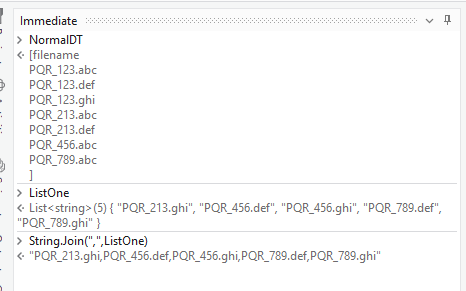# Excel Automation Question - Missing File Report Creation

Hi, Here for each date and number we must have 3 files with extension .abc , .def, .ghi
but sometimes it doesn’t happen and only 2 or only 1 file with either of 3 (.abc, .ghi, .def ) is there for that number.

I want to write all the file names which are missing in a notepad

for e.g. file name PQR_123 has all files but PQR_456 has ghi missing…so I want to write in notepad PQR_456.ghi
Note: PQR is constant, 123/456 here changes but it should be 3 in count ideally containing .abc, .def, .ghiGive a try to this.
FindMissingItem.xaml (12.2 KB)

Hope this helps

1 Like

@anjasing

Please try this in an assign activity the output is a comma separated string…which you can write to text file

`str = String.Join(",",dt.AsEnumerable.GroupBy(function(x) x("ColumnName").ToString.Trim.Split({"."},StringSplitOptions.None)(0)).Where(function(x) x.Count<3).Select(function(x) String.Join(",",{x.Select(function(y) y("ColumnName").ToString)(0).Split({"."},StringSplitOptions.None)(0) + ".abc",x.Select(function(y) y("ColumnName").ToString)(0).Split({"."},StringSplitOptions.None)(0) + ".def",x.Select(function(y) y("ColumnName").ToString)(0).Split({"."},StringSplitOptions.None)(0) + ".ghi"}.Except(x.Select(function(y) y("ColumnName").ToString).ToArray))))`

chnage the columnName as per the columnname you have in your datatable,dt is the input datatable

Hope this helps

cheers

1 Like

Hi @anjasing ,

Check with the below Steps :

1. Assuming you have read the Data as a Datatable, say `NormalDT` using a `Read Range` Activity.
2. Next, Initialise a List of String variable, say `ListOne`.
3. We can then use the below Linq Expression to get the list of values that are not present in the `filename` column using an `Assign` activity :
``````ListOne = (From r In NormalDT
Group By k=Path.GetFileNameWithoutExtension(r("filename").ToString) Into grp=Group
Let NonMatch = {".abc",".def",".ghi"}.Except(grp.Select(Function(y)Path.GetExtension(y("filename").ToString))).ToArray
Select If(NonMatch.Count=0,New List(Of String),NonMatch.Select(Function(x)k+x).ToList)).SelectMany(Function(x)x).ToList
``````
1. Now, We can use this List to get into the string format required using the below Expression :
``````String.Join(",",ListOne)
``````

Implementation :

Debug Visuals :Let us know if you were not able to get the Required output.

2 Likes

Thank you so much for explaining in such a detail!

This topic was automatically closed 3 days after the last reply. New replies are no longer allowed.